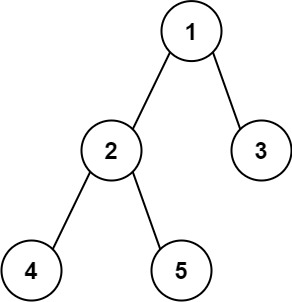# LeetCode: 543. Diameter of Binary Tree

## 题目¶

Given the root of a binary tree, return the length of the diameter of the tree.

The diameter of a binary tree is the length of the longest path between any two nodes in a tree. This path may or may not pass through the root.

The length of a path between two nodes is represented by the number of edges between them.

Example 1:```Input: root = [1,2,3,4,5]
Output: 3
Explanation: 3is the length of the path [4,2,1,3] or [5,2,1,3].
```

Example 2:

```Input: root = [1,2]
Output: 1
```

Constraints:

• The number of nodes in the tree is in the range [1, 10^4].
• -100 <= Node.val <= 100

## 解法¶

```# Definition for a binary tree node.
# class TreeNode:
#     def __init__(self, val=0, left=None, right=None):
#         self.val = val
#         self.left = left
#         self.right = right
class Solution:
def diameterOfBinaryTree(self, root):
self._max_path = 0

self._max_deepth(root)

return self._max_path

def _max_deepth(self, root):
if root is None:
return 0

left_deepth = self._max_deepth(root.left)
right_deepth = self._max_deepth(root.right)

path = left_deepth + right_deepth
if path > self._max_path:
self._max_path = path

return 1 + max(left_deepth, right_deepth)
```